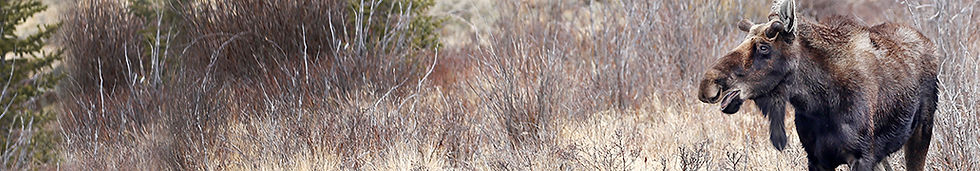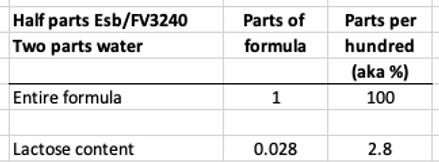# Heavy Whipping Cream Lactose Content

Consider a formula recipe of 1/2 parts each of Esbilac® and FV 32/40 mixed with 2 parts of water, with a fat supplement of an added 1/4 part of heavy whipping cream (HWC). How much does the lactose of the overall volume of formula increase due to the addition of the HWC?

A.) The formula now has an additional 1.0% of lactose,

D.) Some other amount.

How much lactose is in the base formula (without the added HWC)? Easy enough, just input the recipe into WildAgain's Wildlife Formula Calculator and it displays that 2.8% of the formula is carbohydrates. This is mainly lactose except for a few stray molecules. That represents the amount of lactose that is contained in the combination of the two powdered milk replacers.Step 2 ﷯

Now add the 1/4 part HWC. Keep in mind that HWC is mostly fat, and  only about 3% lactose. Input this amended recipe into WildAgain's Nutrition Calculator and it displays that now 2.9% of the formula is carbohydrates. So it did increase in lactose content, but only a tenth of a percent, or .1%.Step 3

Lastly, since most people think in linear terms, they really do not appreciate how relatively small a tenth of a percent really is. So let’s express it another way, in parts per thousand of the entire milk formula (shown left) and we see that the addition of the HWC adds a thousandth of a part of lactose to the entire milk formula.

Using HWC as a fat supplement, as in this example, boosts lactose content by only one-thousandth of a part, but yields the following increases in the formula:

1.) Fat content up 44% with a corresponding increase of kcals of 25%. 2.) For the dietary minerals, concentrations of calcium and phosphorous increase 17% and 23% respectively.

Conclusion

The concern voiced by some that the impact of the use of HWC results in adding a large and unacceptable amount of additional lactose to the formula seems a bit overstated.

For those whose eyes may glaze over with all of the numbers shown above, here is a visual depiction of what a thousandth of a part looks like (without using numbers):